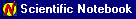Search IntMath
Close

450+ Math Lessons written by Math Professors and Teachers

5 Million+ Students Helped Each Year

1200+ Articles Written by Math Educators and Enthusiasts

Simplifying and Teaching Math for Over 23 Years

# Scientific Notebook InformationI have used Scientific Notebook (SNB) quite a deal when developing this site, especially in the "Advanced Calculus" sections. (For example, see Differential Equations).

Many of the pages were developed in Scientific Notebook, then converted to HTML.

SNB is software that incorporates the MuPAD mathematics engine and a Latex-based word processor. It has awesome power for doing mathematics and for producing text- and math-based documents.

You can get a free trial 30 day download of SNB from here:

Disclaimer: I have no connection with Mackichan Software, makers of SNB.

## Learning Computer Algrebra Skills

It's very important that students learn how to use math software packages. In the "real world", scientists and engineers use packages like MatLab, MathCAD, Maple, Mathematica and Sage to calculate answers to complex problems.

Our students should not only learn basic math skills, but also how to use math software to do more complex analysis.

## Problem SolverThis tool combines the power of mathematical computation engine that excels at solving mathematical formulas with the power of GPT large language models to parse and generate natural language. This creates math problem solver thats more accurate than ChatGPT, more flexible than a calculator, and faster answers than a human tutor. Learn More.